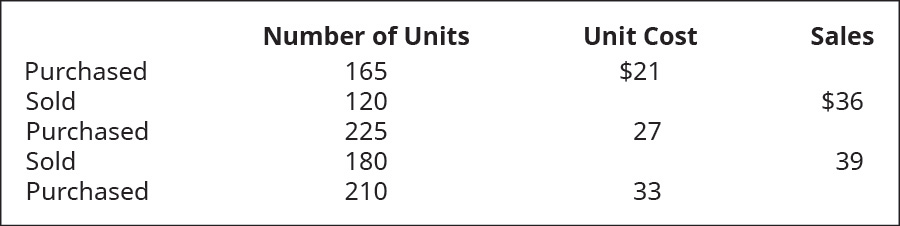# Prepare journal entries to record the following transactions, assuming perpetual inventory updating and first-in, first-out (FIFO) cost allocation. Assume no beginning inventory.FindFindarrow_forward

### Principles of Accounting Volume 1

19th Edition
OpenStax
Publisher: OpenStax College
ISBN: 9781947172685

#### Solutions

Chapter
SectionFindFindarrow_forward

### Principles of Accounting Volume 1

19th Edition
OpenStax
Publisher: OpenStax College
ISBN: 9781947172685
Chapter 10, Problem 8PA
Textbook Problem
1 views

## Prepare journal entries to record the following transactions, assuming perpetual inventory updating and first-in, first-out (FIFO) cost allocation. Assume no beginning inventory.To determine

Introduction:

First In First Out is a method of evaluation of cost of goods sold. In this method, it is assumed that units purchased at first is sold first. Costs in relation with units sold are taken from cost of first lot and in case, the sold units is higher than the first lot purchased, then cost of second lot is used.

To prepare:

Journal entries for the given transactions.

### Explanation of Solution

Record purchase of inventory:

 Date Account Debit ($) Credit($) Inventory (165 units×$21) 3,465 Cash 3,465 (To record purchase of inventory.) Table (1) • Inventory is an asset and it is increased by$3,465. Therefore, Inventory is debited with $3,465. • Cash is an asset and it is decreased by$3,465. Therefore, Cash is credited with $3,465. Record sale of inventory:  Date Account Debit ($) Credit($) Cash (120 units×$36) 4,320 Sales Revenue 4,320 (To record sale of inventory.)

Table (2)

• Cash is an asset and it is increased by $4,320. Therefore, Cash is debited with$4,320.
• Sale is income and it is increased by $4,320. Therefore, Sales Revenue is credited with$4,320.

Record conversion of inventory into COGS:

 Date Account Debit ($) Credit($) COGS (120 units×$21) 2,520 Inventory 2,520 (To record conversion of inventory into COGS.) Table (3) • COGS is an expense and it is increased by$2,520. Therefore, COGS is debited with $2,520. • Inventory is an asset and it is decreased by$2,520. Therefore, Inventory is credited with $2,520. Record purchase of inventory:  Date Account Debit ($) Credit($) Inventory (225 units×$27) 6,075 Cash 6,075 (To record purchase of inventory.)

Table (4)

• Inventory is an asset and it is increased by $6,075. Therefore, Inventory is debited with$6,075.
• Cash is an asset and it is decreased by $6,075. Therefore, Cash is credited with$6,075.

Record sale of inventory:

 Date Account Debit ($) Credit($) Cash (180 units×\$39) 7,020 Sales Revenue 7,020 (To record sale of inventory

### Still sussing out bartleby?

Check out a sample textbook solution.

See a sample solution

#### The Solution to Your Study Problems

Bartleby provides explanations to thousands of textbook problems written by our experts, many with advanced degrees!

Get Started

Find more solutions based on key concepts
What is an XBRL tag?

Accounting Information Systems

PV OF A CASH FLOW STREAM A rookie quarterback is negotiating his first NFL contract. His opportunity cost is 10...

Fundamentals of Financial Management, Concise Edition (with Thomson ONE - Business School Edition, 1 term (6 months) Printed Access Card) (MindTap Course List)

What is the order entry/sales (OE/S) process?

Pkg Acc Infor Systems MS VISIO CD